﻿ 冬奥冰壶六足机器人研究
 机器人2022, Vol. 44Issue (5): 533-5370

GAO Feng, QI Chenkun, CHEN Xianbao, YIN Ke, ZHAO Yue, GAO Yue. On the Six-Legged Curling Robot at the Winter Olympic Games[J]. ROBOT, 2022, 44(5): 533-537.1. 上海交通大学机械系统与振动国家重点实验室，上海 200240;
2. 上海交通大学人工智能研究院教育部人工智能重点实验室，上海 200240

On the Six-Legged Curling Robot at the Winter Olympic Games
GAO Feng1, QI Chenkun1 , CHEN Xianbao1 , YIN Ke2 , ZHAO Yue2 , GAO Yue2
1. State Key Laboratory of Mechanical System and Vibration, Shanghai Jiao Tong University, Shanghai 200240, China;
2. MoE Key Lab of Artificial Intelligence, AI Institute, Shanghai Jiao Tong University, Shanghai 200240, China
Abstract: The six-legged curling robot is the only robot to enter the Winter Olympic Games site required by Beijing Organising Committee for the 2022 Olympic Winter Games. The purpose of developing this robot is to demonstrate the independent innovation ability and level of robots in China at the Beijing Winter Olympic Games. By imitating the behavioural characteristics of a curling player throwing a curling stone, we find unique ideas to design and develop a six-legged curling robot. The design of the six-legged curling robot faces many challenges, including the configuration synthesis of the six-legged robot imitating the human curling behaviour, the establishment of the correlation characteristics between the curling task chain and the robot behaviour chain, and the trajectory planning of the robot hitting the curling stone. The developed six-legged curling robot successfully completed the performance of hitting curling stone at the Ice Cube for the Beijing 2022 Winter Olympic Games.
Keywords: six-legged robot    curling stone    mechanism type    servo driving    robot behaviour control

1 引言（Introduction）

2018年平昌冬奥会后，国际上开始出现轮式有源驱动冰壶机器人研究文章[1-2]。轮式冰壶机器人不是直接模仿人击打冰壶的行为，而是利用轮式机器人的车轮与冰面摩擦驱动冰壶的运动。设计出模仿人投掷冰壶行为的六足机器人，是前人尚未系统研究的科学问题，是一个从无到有的研究过程。模仿人投掷冰壶的行为必须突破多任务、多功能、多末端输出的冰壶机器人设计面临的多项挑战，必须攻克机器人的关键基础部件的核心技术，要解决模仿人投掷冰壶行为的六足机器人的构形综合、投掷冰壶任务链与机器人行为链关联特征建立、机器人击打冰壶行为规划等问题。

2 冰壶六足机器人构形（Configuration of the six-legged curling robot）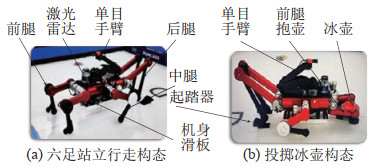图 1 冰壶六足机器人的站立行走和投掷冰壶构态 Fig.1 Configurations of standing and walking, and curling stone throwing of the six-legged curling robot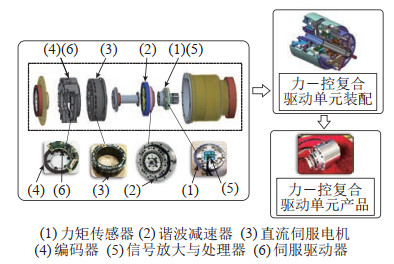图 2 力－控复合驱动单元产品 Fig.2 Product of the force-control composite driving unit
3 冰壶六足机器人行为链（Behaviour chain of the six-legged curling robot）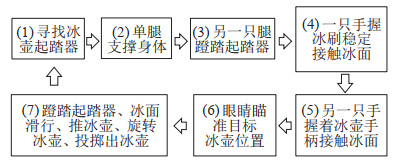图 3 人投掷冰壶的任务链 Fig.3 Task chain of the curling stone delivery by human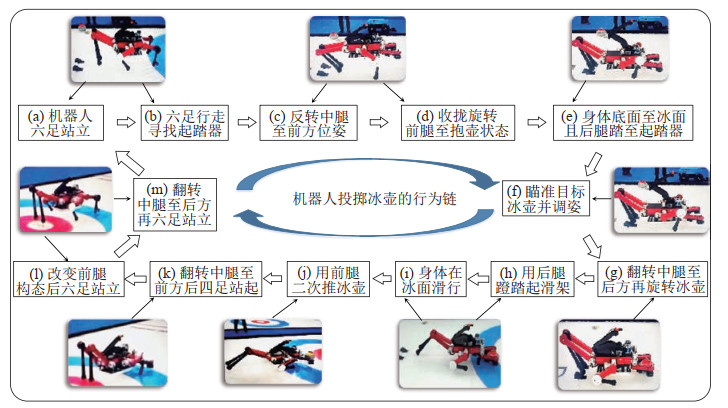图 4 冰壶六足机器人的拟人行为子链分解 Fig.4 Sub-chain decomposition of humanoid behaviours of the six-legged curling robot
4 冰壶六足机器人投壶轨迹规划（Trajectory planning of curling stone delivery by the six-legged curling robot）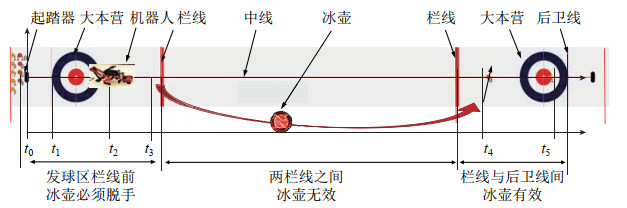图 5 机器人投掷冰壶的场地区域划分 Fig.5 Area division of the curling stone delivery by the robot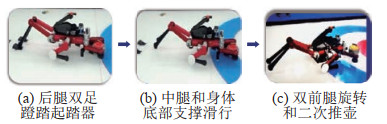图 6 机器人投掷冰壶关键任务行为子链 Fig.6 Behaviour sub-chain of critical tasks of the curling stone delivery by the robot

 \begin{align} q_{j} =\, & c_{0} +c_{1} (t-t_{0})+c_{2} (t-t_{0})^{2}+c_{3} (t-t_{0})^{3} + \\ & c_{4} (t-t_{0})^{4}+c_{5} (t-t_{0})^{5}+c_{6} (t-t_{0})^{6} + \\ & c_{7} (t-t_{0})^{7} \end{align} (1)

 \begin{align} \mathit{\boldsymbol{P}} =\mathit{\boldsymbol{R}}+\mathit{\boldsymbol{r}}, \quad \dot{\mathit{\boldsymbol{P}}} =\dot{\mathit{\boldsymbol{R}}}+\dot{\mathit{\boldsymbol{r}}}, \quad \ddot{\mathit{\boldsymbol{P}}} =\ddot{\mathit{\boldsymbol{R}}}+\ddot{\mathit{\boldsymbol{r}}} \end{align} (2)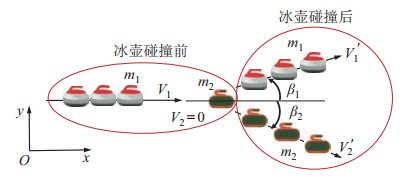图 7 机器人掷壶后冰壶运动 Fig.7 Motion of the curling stone after delivery by the robot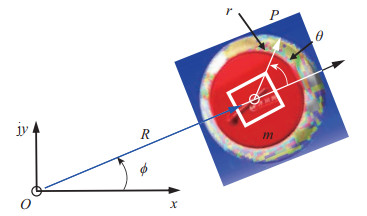图 8 机器人掷壶后冰壶滑行轨迹描述 Fig.8 Trajectory description of the curling stone after delivery by the robot

 \begin{align} \mathit{\boldsymbol{R}} =R{\rm e}^{{\rm j}\phi}, \quad \mathit{\boldsymbol{r}} =r{\rm e}^{{\rm j}(\phi +\theta)} \end{align} (3)

 \begin{equation} \begin{aligned} \dot{\mathit{\boldsymbol{R}}}& =\dot{R}{\rm e}^{{\rm j}\phi} +R\dot{\phi} {\rm e}^{{\rm j} \left(\phi + {\frac{{\rm{ \mathsf{ π} }}} {2}}\right)} \\ \dot{\mathit{\boldsymbol{r}}}& =r({\dot{\phi} +\dot{\theta}}){\rm e}^{{\rm j}\left({\phi +\theta + {\frac{{\rm{ \mathsf{ π} }}} {2}}}\right)} \end{aligned} \end{equation} (4)

 \begin{equation} \begin{aligned} \ddot{\mathit{\boldsymbol{R}}}& =\ddot{R}{\rm e}^{{\rm j}\phi} +2\dot{R}\dot{\phi}{\rm e}^{{\rm j} \left(\phi +\frac{{\rm{ \mathsf{ π} }}} {2}\right)}+R\ddot{\phi} {\rm e}^{{\rm j} \left(\phi +\frac{{\rm{ \mathsf{ π} }}} {2}\right)}+R\dot{\phi}^{2}{\rm e}^{{\rm j}(\phi +{\rm{ \mathsf{ π} }})} \\ \ddot{\mathit{\boldsymbol{r}}}& =r({\ddot{\phi} +\ddot{\theta}}){\rm e}^{{\rm j}({\phi +\theta +\frac{{\rm{ \mathsf{ π} }}} {2}})}+r({\dot{\phi} +\dot{\theta}})^{2}{\rm e}^{{\rm j}({\phi +\theta +{\rm{ \mathsf{ π} }}})} \end{aligned} \end{equation} (5)

 \begin{equation} \begin{aligned} v_{P}^{R} & =\dot{R}+r(\dot{\phi} +\dot{\theta})\cos \left({\theta +\frac{{\rm{ \mathsf{ π} }}} {2}}\right) \\ v_{P}^{T} & =R\dot{\phi} +r(\dot{\phi} +\dot{\theta})\sin \left({\theta +\frac{{\rm{ \mathsf{ π} }}} {2}}\right) \end{aligned} \end{equation} (6)

 \begin{align} \tan \lambda =\frac{v_{P}^{T}} {v_{P}^{R}} \end{align} (7)

 \begin{equation} \begin{aligned} F_{\rm f} & =\sqrt{ \left({F_{\rm f}^{T}}\right)^{2}+ \left({F_{\rm f}^{R}}\right)^{2}} =mgf\\ F_{\rm f}^{R} & =F_{\rm f} \cos \lambda \\ F_{\rm f}^{T} & =F_{\rm f} \sin \lambda \end{aligned} \end{equation} (8)

 \begin{equation} \begin{aligned} \tan \lambda_{1} & =\frac{R\dot{\phi} +r({\dot{\phi}+\dot{\theta}})}{\dot{R}}, \; \; \theta =0 \\ \tan \lambda_{2} & =\frac{R\dot{\phi} -r({\dot{\phi}+\dot{\theta}})}{\dot{R}}, \; \; \theta ={\rm{ \mathsf{ π} }} \end{aligned} \end{equation} (9)

 \begin{align} F_{{\rm f}_{1}} =\frac{1}{2}mgf_{1}, \quad F_{{\rm f}_{2}} =\frac{1}{2}mgf_{2} \end{align} (10)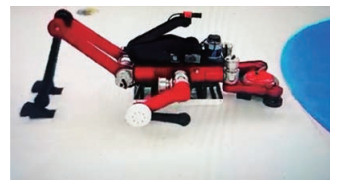图 9 冰壶六足机器人在北京冬奥会掷壶表演 Fig.9 Curling stone throwing show by the six-legged curling robot at the Beijing 2022 Winter Olympic Games
5 后记（Postscript）

  Park H, Oh J H. Robots for the PyeongChang 2018 Winter Olympic Games[J]. Science Robotics, 2018, 3(16). DOI:10.1126/scirobotics.aat5217  Won D O, Müller K R, Lee S W. An adaptive deep reinforcement learning framework enables curling robots with human-like performance in real-world conditions[J]. Science Robotics, 2020, 5(46). DOI:10.1126/scirobotics.abb9764  Tian Y, Gao F. Efficient motion generation for a six-legged robot walking on irregular terrain via integrated foothold selection and optimization-based whole-body planning[J]. Robotica, 2018, 36(3): 333-352.  Gao F, Li S, Gao Y, et al. Robots at the Beijing 2022 Winter Olympics[J]. Science Robotics, 2022, 7(65). DOI:10.1126/scirobotics.abq0785